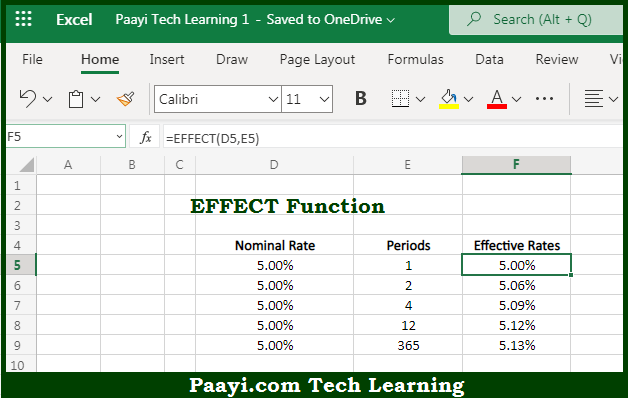# Learn How to Use Microsoft Excel EFFECT Function

Written by | 0 Comments | 570 Views

In this article, you will learn how to use the Microsoft Excel EFFECT function and its prime function in Microsoft Excel. You will also get to know the Microsoft Excel EFFECT function return value and syntax with the help of some examples.

Microsoft Excel EFFECT Function

The main purpose of the Microsoft Excel EFFECT function is to get the effective annual interest rate. That implies, with the help of the EFFECT function you can able to return the effective annual interest rate, given a nominal interest rate and the number of compounding periods per year. Effective annual interest rate is the interest rate actually earned due to compounding. So, with the help of the EFFECT function, you can able to get the effective annual interest rate.

Return Value of EFFECT Function

The return value will be the effective annual interest rate.

Syntax of EFFECT Function

=EFFECT(nominal-rate, npery)

Where the arguments:

• nominal-rate: This is the nominal or stated interest rate.

npery: This is the number of compounding periods per year.

## How to Use Microsoft Excel EFFECT Function?So we know that Microsoft Excel EFFECT function you can able to get get the effective annual interest rate. That implies, with the help of the EFFECT function you can able to return the effective annual interest rate, given a nominal interest rate and the number of compounding periods per year. Effective annual interest rate is the interest rate actually earned due to compounding. So, with the help of the EFFECT function, you can able to get the effective annual interest rate.